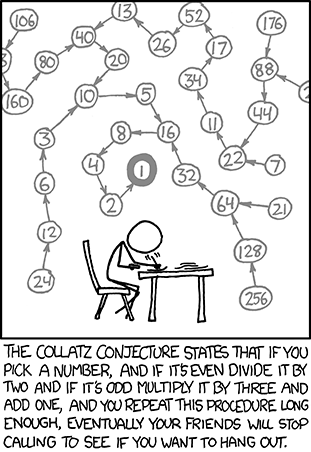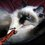# The most difficult - yet so easy to understand - problem in the world!Take any natural number $n$. If $n$ is even, divide it by $2$ to get $n / 2$. If $n$ is odd, multiply it by $3$ and add $1$ to obtain $3n + 1$. Repeat the process indefinitely. The problem is to prove that no matter what number you start with, you will always eventually reach $1$.

Don't be fooled by the simplicity of this problem, great mathematicians like Erdős had great trouble proving it. The latter even said: "Mathematics may not be ready for such problems."Note by حكيم الفيلسوف الضائع
7 years, 2 months ago

This discussion board is a place to discuss our Daily Challenges and the math and science related to those challenges. Explanations are more than just a solution — they should explain the steps and thinking strategies that you used to obtain the solution. Comments should further the discussion of math and science.

When posting on Brilliant:

• Use the emojis to react to an explanation, whether you're congratulating a job well done , or just really confused .
• Ask specific questions about the challenge or the steps in somebody's explanation. Well-posed questions can add a lot to the discussion, but posting "I don't understand!" doesn't help anyone.
• Try to contribute something new to the discussion, whether it is an extension, generalization or other idea related to the challenge.

MarkdownAppears as
*italics* or _italics_ italics
**bold** or __bold__ bold
- bulleted- list
• bulleted
• list
1. numbered2. list
1. numbered
2. list
Note: you must add a full line of space before and after lists for them to show up correctly
paragraph 1paragraph 2

paragraph 1

paragraph 2

[example link](https://brilliant.org)example link
> This is a quote
This is a quote
    # I indented these lines
# 4 spaces, and now they show
# up as a code block.

print "hello world"
# I indented these lines
# 4 spaces, and now they show
# up as a code block.

print "hello world"
MathAppears as
Remember to wrap math in $$ ... $$ or $ ... $ to ensure proper formatting.
2 \times 3 $2 \times 3$
2^{34} $2^{34}$
a_{i-1} $a_{i-1}$
\frac{2}{3} $\frac{2}{3}$
\sqrt{2} $\sqrt{2}$
\sum_{i=1}^3 $\sum_{i=1}^3$
\sin \theta $\sin \theta$
\boxed{123} $\boxed{123}$

Sort by:

When do you predict that the Collatz conjecture will be solved? @Sharky Kesa @Daniel Liu @Daniel Chiu @Trevor B.

- 7 years, 2 months ago

Possibly in the next decade given the number of young mathematicians rising up and are being seen on Brilliant.

- 7 years, 2 months ago

The Collatz Conjecture? or The Ulam's Conjecture?

- 7 years, 2 months ago

It's commonly named after Collatz name but it is also named Ulam's conjecture because he used to talk about it in the lectures he gave.

I did not understand what were you saying about odd numbers. Even one is true but can you explain me the odd process ?

- 7 years, 2 months ago

Start with any natural number. If the number is even, divide it by two. If it is odd, triple it and add one. Repeat the process with the new number so formed. Ultimately the number 1 is reached.

- 7 years, 2 months ago

Even one is true. But what about odd ? Let the no. be 5. if we triple it and add one, we will get 16 and then 49 then 148 ......... Which I don't think would approach 1. Or am I not getting the process. Can u explain the process with examples.

- 7 years, 2 months ago

When you got 16, you'll have to use the operation we restricted to even numbers, that is: $n/2$. And so you will get 8. Repeat it again you'll have 4, then 2, then 1!

OK. Now I got it very well. Thanks

- 7 years, 2 months ago

You're welcome!

You have done the operation wrong. Lets call the operation C(x) where x is your starting number.

$C(5) = 16, C(16) = 8, C(8) = 4, C(4) = 2, C(2) = 1$. And thus the operation ends.

- 7 years, 2 months ago

I also thought this ....one day....Thanks for posting it

- 7 years, 2 months ago

couldn't we just consider it true until proven wrong? =)

- 7 years, 2 months ago

That wouldn't be helpful in any way.

Yep, just taking the lazy way out.

- 7 years, 1 month ago

Wouldn't it be correct if I say it will also reach every time reach 2 .

- 7 years, 2 months ago

No. If you start on 2 itself, then you will have to carry out the operation n/2 anyway. You have to COMPLETE the sequence and hence end with 1.

- 7 years, 1 month ago

But by starting with 2 , 2 comes in our sequence. How will you write the sequence - only 1 or 2 , 1. Consider the following example - We take n = 5 .Then how would you write the sequence like this - 5 , 16 , 8 , 4 , 2 , 1 or simply ignoring 5, by - 16, 8 , 4 , 2 , 1. Of course first one 's correct so we could never ever find a sequence except n = 1 in which two does not come.

- 7 years, 1 month ago

Le problème de Syracuse ;)

- 7 years, 2 months ago